NEET  >  NEET Previous Year Questions (2014-22): Alternating Current

# NEET Previous Year Questions (2014-22): Alternating Current - Notes | Study Physics Class 12 - NEET

 1 Crore+ students have signed up on EduRev. Have you?

Q.1. A series LCR circuit with inductance 10 H, capacitance 10 μF , resistance 50 Ω is connected to an ac source of voltage, V = 200sin(100t) volt. If the resonant frequency of the LCR circuit is  νand the frequency of the ac source is ν , then               (2022)
(1)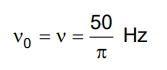(2)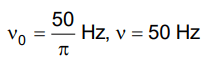(3)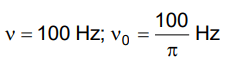(4)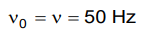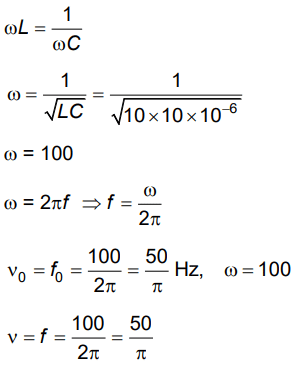Q.2. The peak voltage of the ac source is equal to        (2022)
(1) The rms value of the ac source
(2) √2 times the rms value of the ac source
(3) 1/√2 times the rms value of the ac source

(4) The value of voltage supplied to the circuit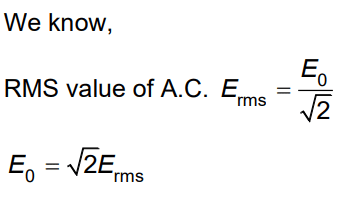Q.3. An inductor of inductance L, a capacitor of capacitance C and a resistor of resistance 'R' are connected in series to an ac source of potential difference 'V' volts as shown in fig. Potential difference across L, C and R is 40 V, 10 V and 40V, respectively. The amplitude of the current flowing through LCR series circuit is 10√2A. The impedance of the circuit is :-     (2021)
A: 4Ω

B: 5Ω
C: 4√2Ω
D: 5√2Ω

Ans: B
Solution:

I0 = 10√2 AQ.4. A step down transformer connected to an ac mains supply of 220 V is made to operate at 11 V, 44 W lamp. Ignoring power losses in the transformer, what is the current in the primary circuit?     (2021)
A: 2 A

B: 4 A
C: 0.2 A
D: 0.4 A
Ans:
C
Solution: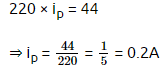Q.5. A series LCR circuit containing 5.0 H inductor, 80 μF capacitor and 40 Ω resistor is connected to 230 V variable frequency ac source. The angular frequencies of the source at which power transferred to the circuit is half the power at the resonant angular frequency are likely to be      (2021)

Ans:
A
Solution:

Q.6. A series LCR circuit is connected to an AC voltage source. When L is removed from the circuit, the phase difference between current and voltage is π/3. If instead C is removed from the circuit, the phase difference is again π/3 between current and voltage. The power factor of the circuit is :     (2020)
A: 1.0
B: –1.0
C: zero
D: 0.5
Ans:
A
Solution:
When inductor alone is removed,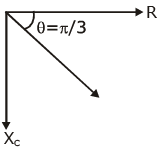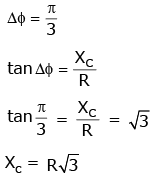When capacitor alone is removed,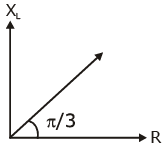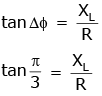Thus, for the original circuit,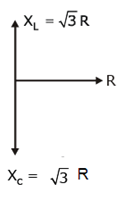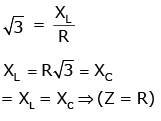power factor = cos Δϕ = R/Z = R/R = 1

Q.7. Which of the following acts as a circuit protection device?    (2019)
A: Conductor
B: Inductor
C: Switch
D: Fuse
Ans:
D
Solution:

Fuse wire has less melting point so when excess current  flows, due to heat produced in it, it melts.

Q.8. A parallel plate capacitor of capacitance 20 mF is being charged by a voltage source whose potential is changing at the rate of 3 V/s. The conduction current through the connecting wires, and the displacement current through the plates of the capacitor, would be, respectively.    (2019)
A: Zero, 60 μA
B: 60 μA, 60 μA
C: 60 μA, zero
D: Zero, zero
Ans:
B
Solution:

Capacitance of capacitor C = 20 mF
= 20 × 10-6 F
Rate of change of potential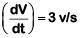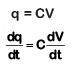ic = 20 × 10–6 × 3
= 60 × 10–6 A
= 60 μA
As we know that id = ic = 60 mA

Q.9. The magnetic potential energy stored in a certain inductor is 25 mJ, when the current in the inductor is 60 mA. This inductor is of inductance :-    (2018)
A: 0.138 H
B: 138.88 H
C: 1.389 H
D: 13.89 H
Ans:
D
Solution:

Energy stored in the inductor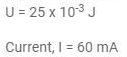Energy stored in the inductor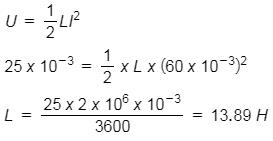Q.10. In the circuit shown in the figure, the input voltage Vi is 20 V, VBE = 0 and VCE = 0. The values of IB, IC and β are given by :-    (2018)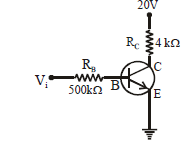A: IB = 40 µA, IC = 10 mA, β = 250
B: IB = 25 µA, IC = 5 mA, β = 200
C: IB = 20 µA, IC = 5 mA, β = 250
D: IB = 40 µA, IC = 5 mA, β = 125
Ans:
D
Solution: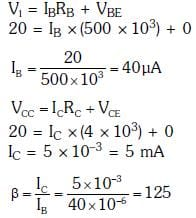Q.11. An inductor 20 mH, a capacitor 100 μF and a resistor 50 Ω are connected in series across a source of emf, V = 10 sin 314 t. The power loss in the circuit is     (2018)
A: 0.79 W
B: 0.43 W
C: 2.74 W
D: 1.13 W
Ans:
A
Solution: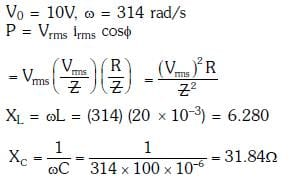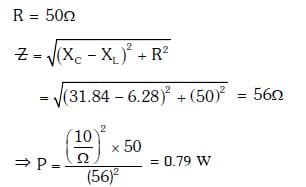Q.12. Figure shows a circuit that contains three identical resistors with resistance R = 9.0 Ω each, two identical inductors with inductance L = 2.0 m Heach, and an ideal battery with emf ε = 18 V. The current 'i' through the battery just after the switch closed is,...... :-      (2017)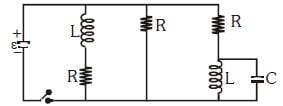A: 0.2 A
B: 2 A
C: 0 ampere
D: 2 mA
Ans:
B
Solution: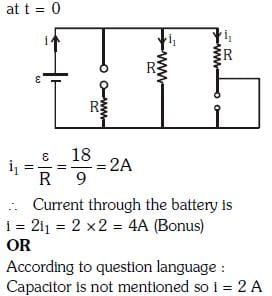Q.13. An inductor 20 mH, a capacitor 50 μF and a resistor. 40Ω are connected in series across a source of emf V = 10 sin 340 t. The power loss in A.C. circuit is     (2016)
A: 0.89W
B: 0.51W
C: 0.67W
D: 0.76W
Ans:
B
Solution: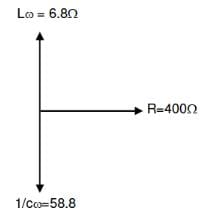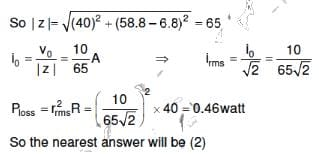Q.14. A small signal voltage V(t) = V0 sinωt is applied across an ideal capacitor C :    (2016)
A: Current I(t), leads voltage V(t) by 180 degree
B: Current I(t), lags voltage V(t) by 90 degree
C: Over a full cycle the capacitor C does not consume any energy from the voltage source.
D: Current I(t) is in phase with voltage V(t)
Ans:
C
Solution:

Capacitor does not consume energy effectively over full cycles

Q.15. A resistance 'R' draws power 'P' when connected to an AC source. If an inductance is now placed in series with the resistance, such that the impedance of the circuit becomes 'Z', the power drawn will be :
A: P
B: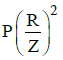C: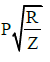D: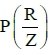Ans: B
Solution:

A resistance R draws power P when connected to an AC source.
The magnitude of voltage of the AC source is
V2 = RP
∴ V=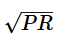An inductor of inductance L and reactance ωL is now placed in series with the resistance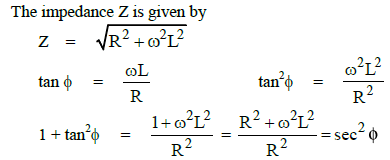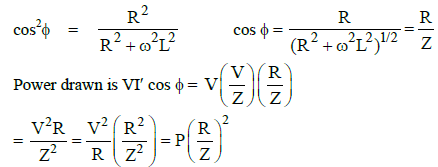Q.16. A transformer having efficiency of 90% is working on 200 V and 3 kW power supply. If the current in the secondary coil is 6A, the voltage across the secondary coil and the current in the primary coil respectively are :    (2014)
A: 450 V, 13.5 A
B: 600 V, 15 A
C: 300 V, 15 A
D: 450 V, 15 A

Ans: D
Solution:
Initial power = 3000 W
As the efficiency is 90%, then final power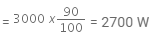That is,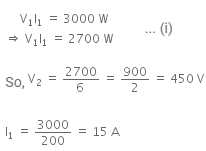The document NEET Previous Year Questions (2014-22): Alternating Current - Notes | Study Physics Class 12 - NEET is a part of the NEET Course Physics Class 12.
All you need of NEET at this link: NEET

## Physics Class 12

157 videos|452 docs|213 tests
 Use Code STAYHOME200 and get INR 200 additional OFF

## Physics Class 12

157 videos|452 docs|213 tests

### How to Prepare for NEET

Read our guide to prepare for NEET which is created by Toppers & the best Teachers

Track your progress, build streaks, highlight & save important lessons and more!

,

,

,

,

,

,

,

,

,

,

,

,

,

,

,

,

,

,

,

,

,

;